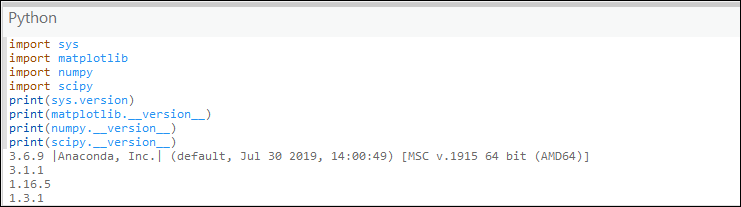# What version of Python is used in ArcGIS?

Last Published: April 19, 2023

The Python programming language has been used in each version since ArcGIS 9.0. It is incorporated into the setups of ArcGIS Desktop, ArcGIS Pro, and ArcGIS Enterprise. ArcGIS installs the versions of Python listed below.

The version of NumPy and Matplotlib is included with the Python environment in the most recent releases.

```Note:
It is not recommended to install an independent version of Python for use in ArcGIS Desktop. Using a different version of Python can lead to compatibility issues and root folder problems. It is not supported to replace the version of Python that ships with ArcGIS Server.```

ArcGIS Pro

The version of Python and its stack versions can be determined by opening the Python window in the application by clicking View > Python, and typing the following commands:

```import sys
import matplotlib
import numpy
import scipy
print(sys.version)
print(matplotlib.__version__)
print(numpy.__version__)
print(scipy.__version__)```

The commands display the default Python version used with the application. In the image below (ArcGIS Pro 2.5), the version of Python is 3.6.9, followed by the versions of the Matplotlib, NumPy, and SciPy packages. Many other packages are also included with the Python environment. Use the Python tab in the ArcGIS Pro settings to check the version of those packages .```Note:
In ArcGIS Pro versions 1.0 through 1.2, if Python is used outside of ArcGIS Pro, it is required to install Python for ArcGIS Pro. This is not required for versions 1.3 and later.```

ArcGIS Desktop (ArcMap)

 ArcGIS Desktop Versions Python and NumPy Versions 10.8.2 Python 2.7.18.4 and NumPy 1.16.6 10.8.1 Python 2.7.18 and NumPy 1.16.6 10.8 Python 2.7.18 and NumPy 1.16.6 10.7.1 Python 2.7.16 and NumPy 1.9.3 10.7 Python 2.7.15 and NumPy 1.9.3 10.6.1 Python 2.7.14 and NumPy 1.9.3 10.6 Python 2.7.14 and NumPy 1.9.3 10.5.1 Python 2.7.13 and NumPy 1.9.3 10.5 Python 2.7.12 and NumPy 1.9.3 10.4.x Python 2.7.10 and NumPy 1.9.2 10.3.x Python 2.7.8 and NumPy 1.7.1 10.2.1 Python 2.7.5 and NumPy 1.7.1 10.2.2 Python 2.7.5 and NumPy 1.7.1 10.2 Python 2.7.3 and NumPy 1.6.1 10.1 Python 2.7.2 and NumPy 1.6.1 10.0 Python 2.6.5 and NumPy 1.3.0

ArcGIS Enterprise

ArcGIS Enterprise also ships with Python. In the latter releases of ArcGIS Enterprise 10.x, both Python 2.x and Python 3.x runtimes are included with the ArcGIS Server component. Beginning with ArcGIS Enterprise 11.0, only Python 3.x is included.

 ArcGIS Enterprise Versions Python, NumPy, and Matplotlib Versions 11.1 Python 3.9.16, NumPy 1.20.1, and matplotlib 3.6.0 11.0 Python 3.9.11, NumPy 1.20.1, and matplotlib 3.4.3 10.9.1 Python 2.7.18.4, NumPy 1.16.6, and matplotlib 2.2.5 10.9.1 Python 3.7.11, NumPy 1.20.1, and matplotlib 3.4.2 10.9 Python 2.7.18, NumPy 1.9.3, and matplotlib 1.5.2 10.9 Python 3.7.9, NumPy 1.19.1, and matplotlib 3.3.1 10.8.1 Python 2.7.18, NumPy 1.9.3, and matplotlib 1.5.2 10.8.1 Python 3.6.10, NumPy 1.18.1, and matplotlib 3.2.1 10.8 Python 2.7.16, NumPy 1.9.3, and matplotlib 1.5.2 10.8 Python 3.6.9, NumPy 1.16.5, and matplotlib 3.1.1 10.7.1 Python 2.7.16, NumPy 1.9.3, and matplotlib 1.5.2 10.7.1 Python 3.6.8, NumPy 1.16.2, matplotlib 3.0.3 10.7 Python 2.7.15, NumPy 1.9.3, and matplotlib 1.5.2 10.7 Python 3.6.6, NumPy 1.15.1, matplotlib 2.2.3 10.6.1 Python 2.7.14, NumPy 1.9.3, and matplotlib 1.5.2 10.6.1 Python 3.6.5, NumPy 1.14.2, matplotlib 2.2.2 10.6 Python 2.7.14, NumPy 1.9.3, and matplotlib 1.5.2 10.6 Python 3.6.2 10.5.1 Python 2.7.13, NumPy 1.9.3, and matplotlib 1.5.2 10.5.1 Python 3.5.3 10.5 Python 2.7.12, NumPy 1.9.3, and matplotlib 1.5.2 10.5 Python 3.5.2 10.4 and 10.4.1 Python 2.7.10, NumPy 1.9.2, and matplotlib 1.4.3 10.4 and 10.4.1 Python 3.4.1 10.3.x Python 2.7.8, NumPy 1.7.1, and matplotlib 1.3.0 10.2.1 and 10.2.2 Python 2.7.5 and NumPy 1.7.1 10.2 Python 2.7.3 and NumPy 1.6.1 10.1 Python 2.7.2 and NumPy 1.6.1 10.0 Python 2.6.5 and NumPy 1.3.0

ArcGIS Notebook Server

Each notebook runtime in ArcGIS Notebook Server packages a precise list of Python libraries, including a specific version of each. If you need a library that is not in either runtime by default, you can extend a notebook runtime to include it. Refer to ArcGIS Notebook Server: Available Python libraries for a complete list of the Python libraries packaged in each default runtime.

Article ID:000013224

Software:
• ArcGIS Server
• ArcGIS Pro
• ArcMap

Get help from ArcGIS experts

Contact technical support